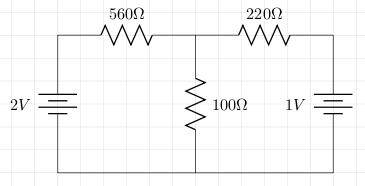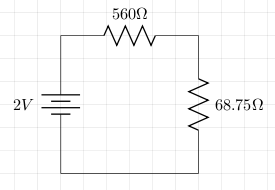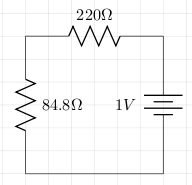# Superposition

The superposition theorem states that in a circuit containing several EMF sources, the current at any branch is the sum of currents at that branch that would be produced by each EMF source acting alone and all other sources of EMF were replaced by their internal resistance.

## Example

We will use superposition to calculate the current through the 100 ohm resistor in the following circuit:We first short the 1V battery and calculate the resistance of the 220 ohm and 100 ohm resistors in parallel which gives 68.75 ohms. We assume the battery has no internal resistance to keep things simple. This gives us the following circuit:We then calculate the current which is 2/(560+68.75) = 3.18×10-3. The voltage across the 68.75 ohm resistor is 3.18×10-3 × 68.75 = 0.218V. This means that the current through the 100 ohm resistor is 0.218/100 = 2.18×10-3A

We then short the 2V battery and calculate the resistance of the 560 ohm and 100 ohm resistors in parallel which gives 84.8 ohms. This gives us the following circuit.We then calculate the current which is 1/(220+84.8) = 3.28×10-3. The voltage across the 84.8 ohm resistor is 3.28×10-3 × 84.8 = 0.278V. This means that the current through the 100 ohm resistor is 0.278/100 = 2.78×10-3A

We now add up all the currents to give 2.18×10-3 + 2.78×10-3 = 4.96 ×10-3A.

When the circuit was built the voltage across the 100 ohm resistor was measured at 0.5V which is very close to the calculated 0.496V (100 × 4.96 ×10-3).

## References

Fischer-Cripps. A.C., The Electronics Companion. Institute of Physics, 2005.